Home > ACC7 > Chapter cc38 > Lesson cc38.2.3 > Problem8-96

8-96.
1. Simplify each expression. Homework Help ✎

1.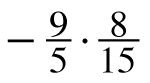2.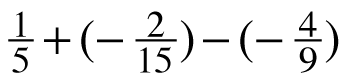3.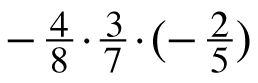4.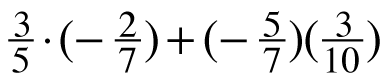5.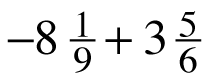6.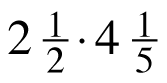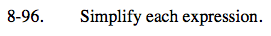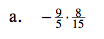Multiply the numerators together and the denominators together.

$-\frac{9(8)}{5(15)} = -\frac{72}{75}$

$-\frac{24}{25}$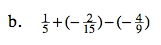Find a common denominator.

$\frac{9}{45}+\left(-\frac{6}{45}\right)-\left(-\frac{20}{45}\right)$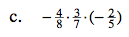Multiply, see (a) for help.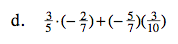Multiply the terms, then find the common denominator.

$-\frac{27}{70}$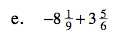Make fractions greater than one, then find the least common denominator.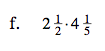Convert the mixed numbers into fractions greater than one before multiplying.

$10\frac{1}{2}$